Publicité

# morphological image processing

13 Jun 2013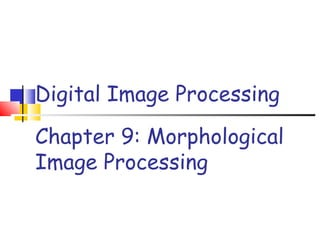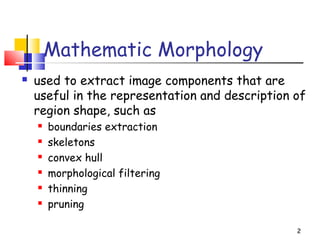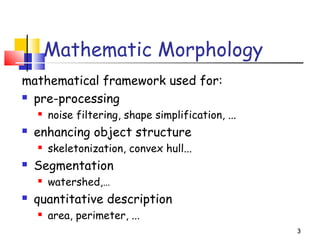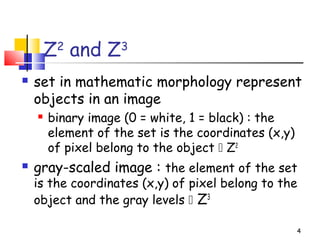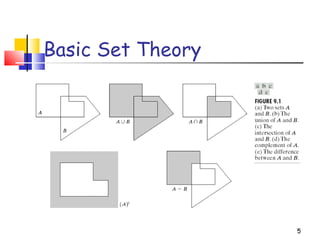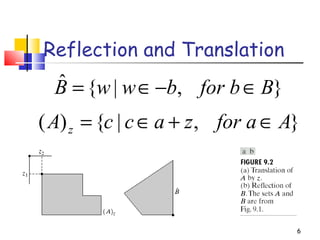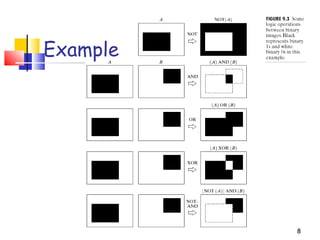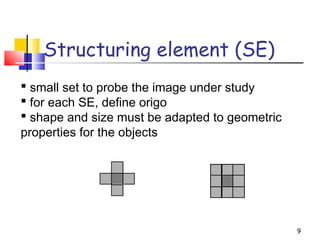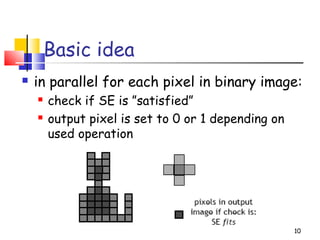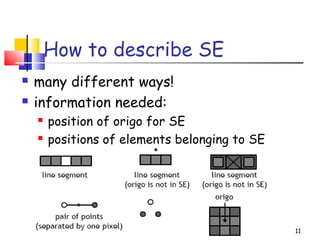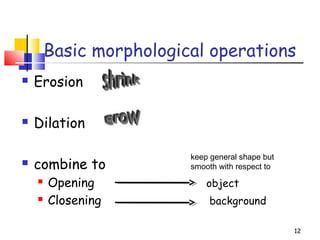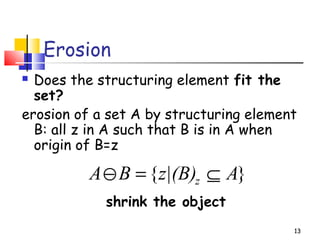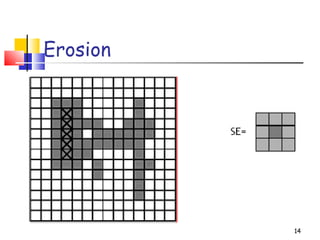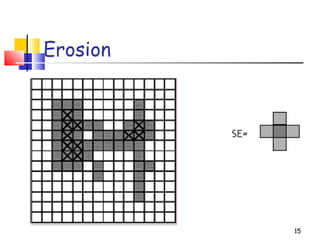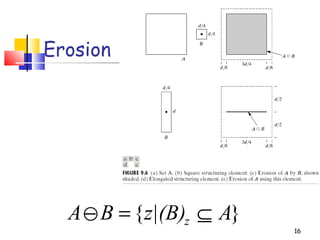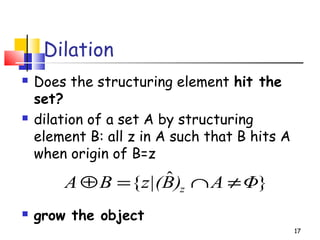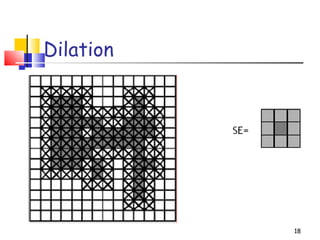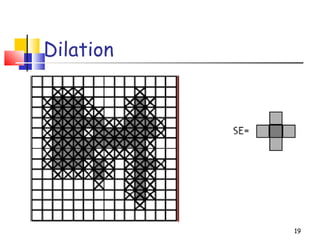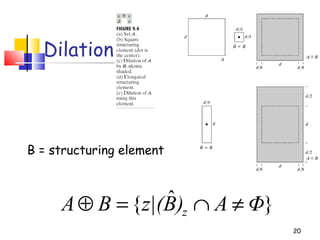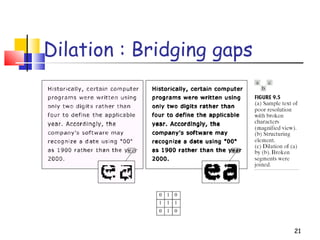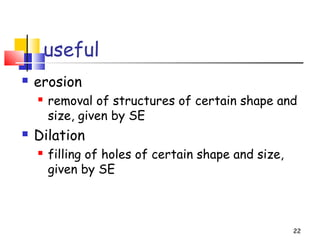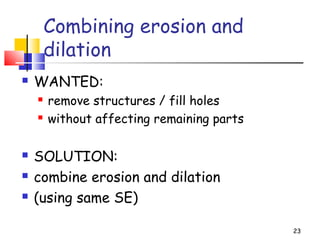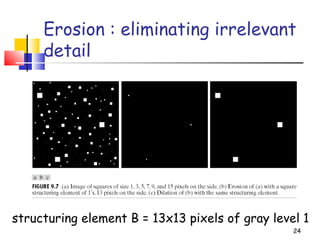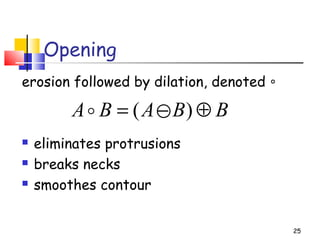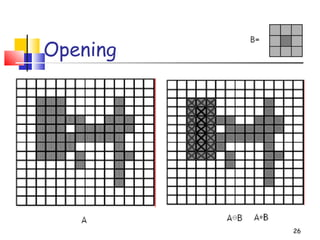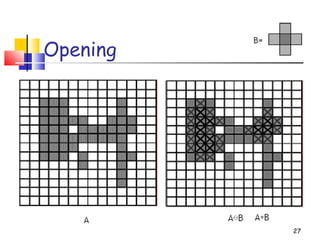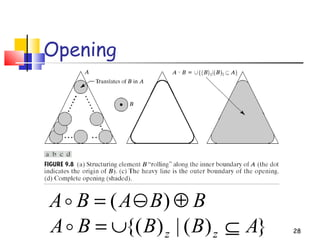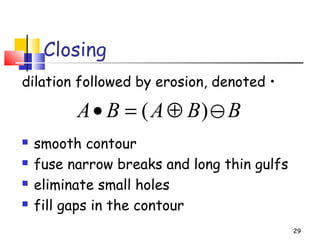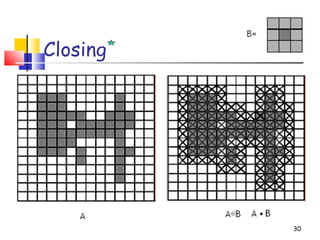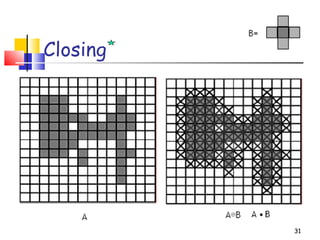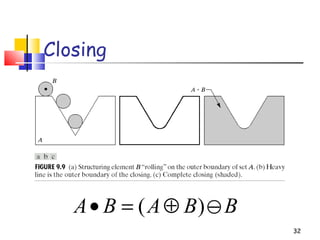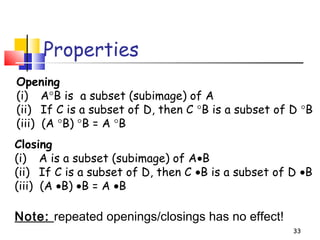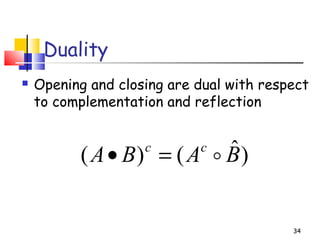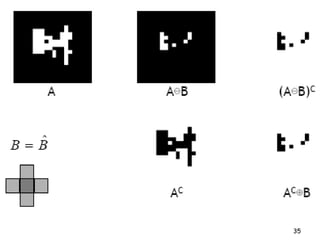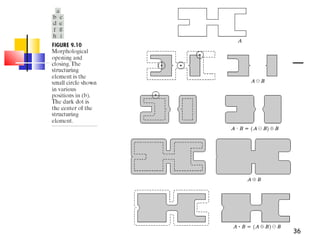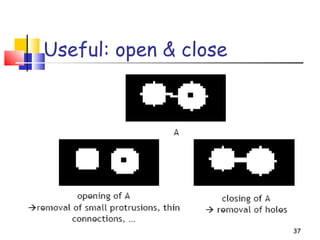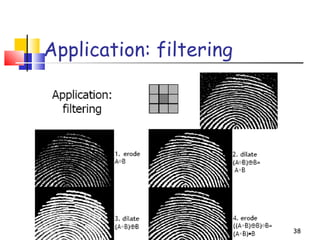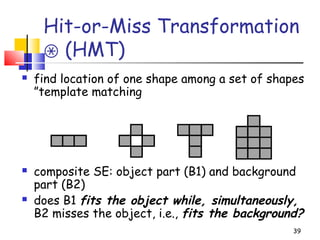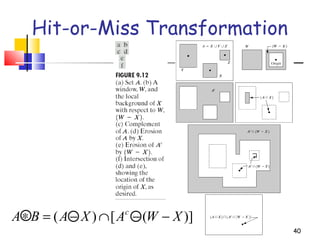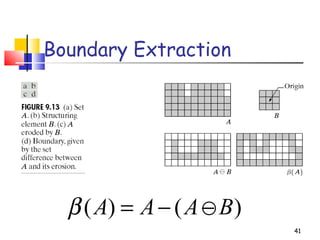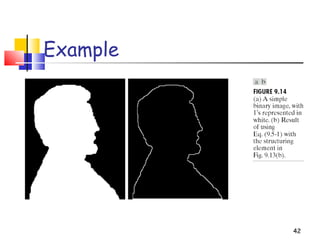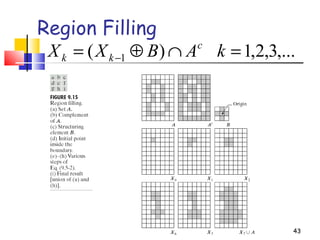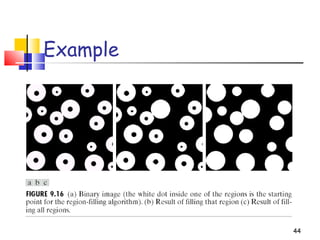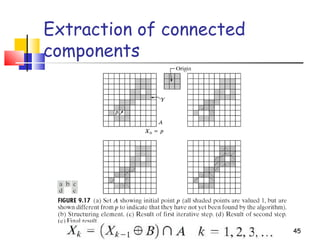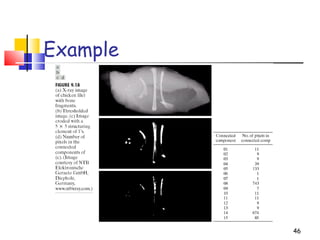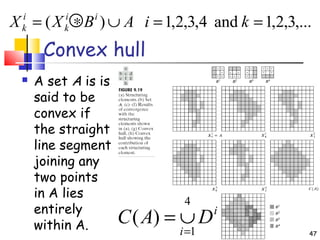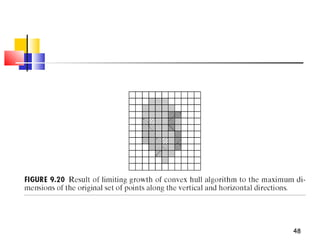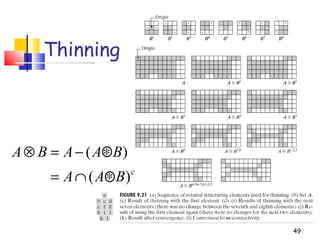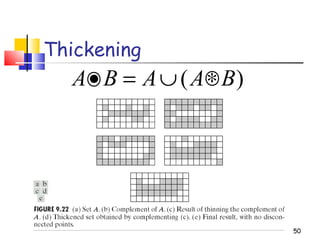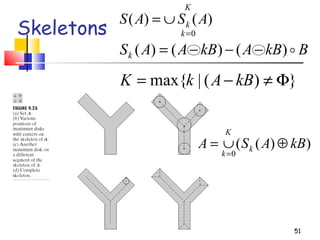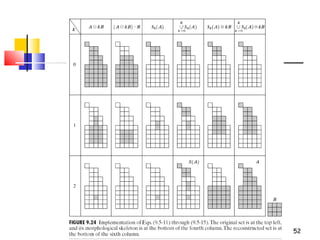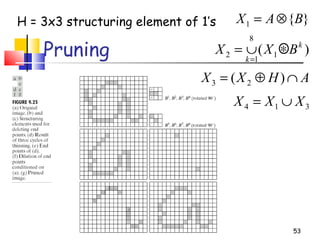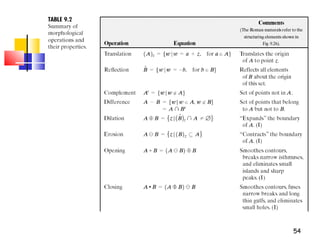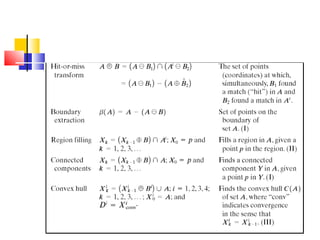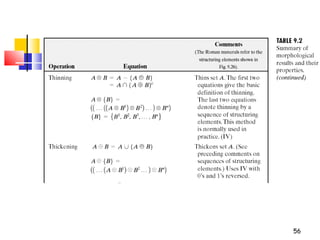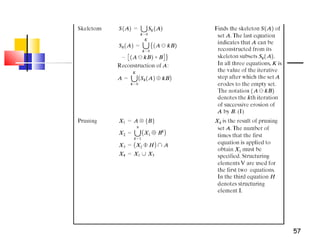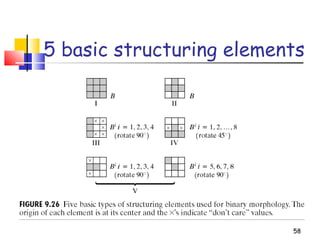1 sur 58
Publicité

### morphological image processing

1. Chapter 9: Morphological Image Processing Digital Image Processing
2. 2 Mathematic Morphology  used to extract image components that are useful in the representation and description of region shape, such as  boundaries extraction  skeletons  convex hull  morphological filtering  thinning  pruning
3. 3 Mathematic Morphology mathematical framework used for:  pre-processing  noise filtering, shape simplification, ...  enhancing object structure  skeletonization, convex hull...  Segmentation  watershed,…  quantitative description  area, perimeter, ...
4. 4 Z2 and Z3  set in mathematic morphology represent objects in an image  binary image (0 = white, 1 = black) : the element of the set is the coordinates (x,y) of pixel belong to the object  Z2  gray-scaled image : the element of the set is the coordinates (x,y) of pixel belong to the object and the gray levels  Z3
5. 5 Basic Set Theory
6. 6 Reflection and Translation },|{ˆ Bfor bbwwB ∈−∈= },|{)( Afor azaccA z ∈+∈=
7. 7 Logic Operations
8. 8 Example
9. Structuring element (SE) 9  small set to probe the image under study  for each SE, define origo  shape and size must be adapted to geometric properties for the objects
10. Basic idea  in parallel for each pixel in binary image:  check if SE is ”satisfied”  output pixel is set to 0 or 1 depending on used operation 10
11. How to describe SE  many different ways!  information needed:  position of origo for SE  positions of elements belonging to SE 11
12. Basic morphological operations  Erosion  Dilation  combine to  Opening object  Closening background 12 keep general shape but smooth with respect to
13. Erosion  Does the structuring element fit the set? erosion of a set A by structuring element B: all z in A such that B is in A when origin of B=z shrink the object 13 }{ Az|(B)BA z ⊆=−
14. Erosion 14
15. Erosion 15
16. 16 Erosion }{ Az|(B)BA z ⊆=−
17. Dilation  Does the structuring element hit the set?  dilation of a set A by structuring element B: all z in A such that B hits A when origin of B=z  grow the object 17 }ˆ{ ΦA)Bz|(BA z ≠∩=⊕
18. Dilation 18
19. Dilation 19
20. 20 Dilation }ˆ{ ΦA)Bz|(BA z ≠∩=⊕ B = structuring element
21. 21 Dilation : Bridging gaps
22. useful  erosion  removal of structures of certain shape and size, given by SE  Dilation  filling of holes of certain shape and size, given by SE 22
23. Combining erosion and dilation  WANTED:  remove structures / fill holes  without affecting remaining parts  SOLUTION:  combine erosion and dilation  (using same SE) 23
24. 24 Erosion : eliminating irrelevant detail structuring element B = 13x13 pixels of gray level 1
25. Opening erosion followed by dilation, denoted ∘  eliminates protrusions  breaks necks  smoothes contour 25 BBABA ⊕−= )(
26. Opening 26
27. Opening 27
28. 28 Opening BBABA ⊕−= )( })(|){( ABBBA zz ⊆∪=
29. Closing dilation followed by erosion, denoted •  smooth contour  fuse narrow breaks and long thin gulfs  eliminate small holes  fill gaps in the contour 29 BBABA −⊕=• )(
30. Closing 30
31. Closing 31
32. 32 Closing BBABA −⊕=• )(
33. 33 Properties Opening (i) A°B is a subset (subimage) of A (ii) If C is a subset of D, then C °B is a subset of D °B (iii) (A °B) °B = A °B Closing (i) A is a subset (subimage) of A•B (ii) If C is a subset of D, then C •B is a subset of D •B (iii) (A •B) •B = A •B Note: repeated openings/closings has no effect!
34. Duality  Opening and closing are dual with respect to complementation and reflection 34 )ˆ()( BABA cc =•
35. 35
36. 36
37. Useful: open & close 37
38. Application: filtering 38
39. Hit-or-Miss Transformation (HMT)⊛  find location of one shape among a set of shapes ”template matching  composite SE: object part (B1) and background part (B2)  does B1 fits the object while, simultaneously, B2 misses the object, i.e., fits the background? 39
40. 40 Hit-or-Miss Transformation )]([)( XWAXABA c −−∩−=∗
41. 41 Boundary Extraction )()( BAAA −−=β
42. 42 Example
43. 43 Region Filling ,...3,2,1)( 1 =∩⊕= − kABXX c kk
44. 44 Example
45. 45 Extraction of connected components
46. 46 Example
47. Convex hull  A set A is is said to be convex if the straight line segment joining any two points in A lies entirely within A. i i DAC 4 1 )( = ∪= ,...3,2,1and4,3,2,1)( ==∪∗= kiABXX ii k i k 47
48. 48
49. 49 Thinning c BAA BAABA )( )( ∗∩= ∗−=⊗
50. 50 Thickening )( BAABA ∗∪=•
51. 51 Skeletons K k k ASAS 0 )()( = ∪= BkBAkBAASk )()()( −−−= })(|max{ Φ≠−= kBAkK ))(( 0 kBASA k K k ⊕∪= =
52. 52
53. 53 Pruning }{1 BAX ⊗= AHXX ∩⊕= )( 23 314 XXX ∪= H = 3x3 structuring element of 1’s )( 1 8 1 2 k k BXX ∗∪= =
54. 54
55. 55
56. 56
57. 57
58. 58 5 basic structuring elements

### Notes de l'éditeur

1. Department of Computer Engineering, CMU
Publicité